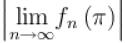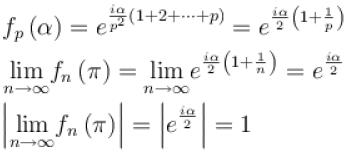Courses

# Complex Number NAT Level - 2

## 10 Questions MCQ Test Topic wise Tests for IIT JAM Physics | Complex Number NAT Level - 2

Description
This mock test of Complex Number NAT Level - 2 for IIT JAM helps you for every IIT JAM entrance exam. This contains 10 Multiple Choice Questions for IIT JAM Complex Number NAT Level - 2 (mcq) to study with solutions a complete question bank. The solved questions answers in this Complex Number NAT Level - 2 quiz give you a good mix of easy questions and tough questions. IIT JAM students definitely take this Complex Number NAT Level - 2 exercise for a better result in the exam. You can find other Complex Number NAT Level - 2 extra questions, long questions & short questions for IIT JAM on EduRev as well by searching above.
*Answer can only contain numeric values
QUESTION: 1

### If perimeter of the locus represented by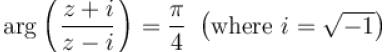is  k,  then find the value of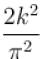Solution: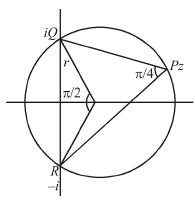r2 + r2 = 4
⇒ r =  √2
perimeter = 3/2 πr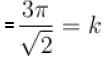*Answer can only contain numeric values
QUESTION: 2

### If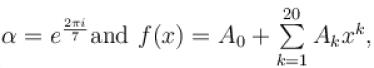then the value of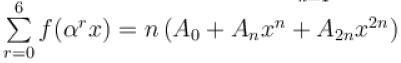then find the value of  n.

Solution:

∴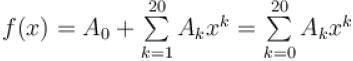∴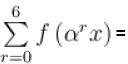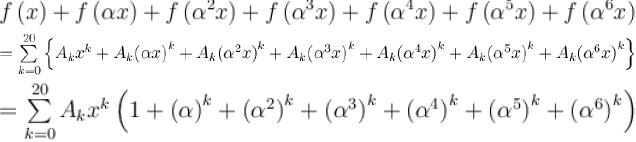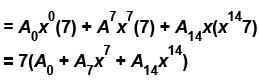n = 7

*Answer can only contain numeric values
QUESTION: 3

### If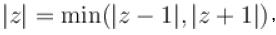then find the value of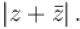Solution:

If    |z| = |z – 1|
⇒ |z|2 = |z – 1|2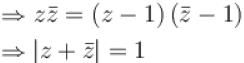again if  |z| = |z + 1|
⇒ |z|2 = |z + 1| 2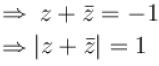*Answer can only contain numeric values
QUESTION: 4

If  z is a complex number and the minimum value of |z| + |z - 1| + |2z - 3|  is λ and if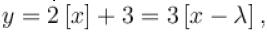then find the value of [x + y].  (where [·] denotes the greatest integer function)

Solution:

∴ |z| + |z – 1| + |2z – 3| =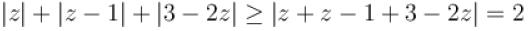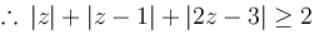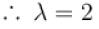then  2[x] + 3 =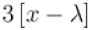= 3[x – 2]
⇒ 2[x] + 3 = 3([x] – 2)
or  [x] = 9
then     y = 2·9 + 3 = 21
∴ [x + y] = [x + 21] = [x] + 21 = 9 + 21 = 30

*Answer can only contain numeric values
QUESTION: 5

If |z2 + iz1 | = |z1 | + |z2 | and  |z1 | = 3 and |z | = 4 then area of Δ ABC, if affix of A, B  and  C  are (z1 ), (z2 ) and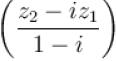respectively is :

Solution: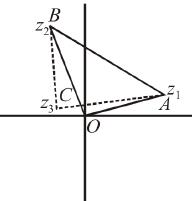+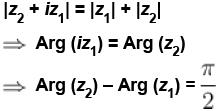Let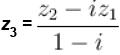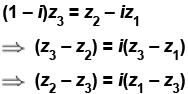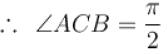and |z2– z3 | = |z1 – z3 |
⇒ AC = BC
∴ AB2 = AC2 + BC2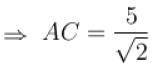∴ (AB =5)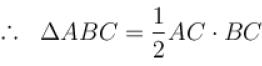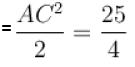square unit

*Answer can only contain numeric values
QUESTION: 6

Find the number of solutions of the equation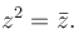Solution:

Let     z = x + iy
⇒ x2 – y2 + 2ixy = x – iy
⇒ x2 – y2 = x
and
2xy = –y
From (2),
2xy = –y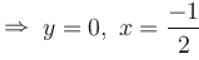When y = 0 from (1), we get
x = 0, 1
when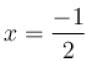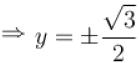Hence the solution of the equation are
z1 = 0 + 1.0, z2 = 1 + 1.0 = 1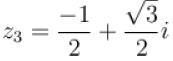and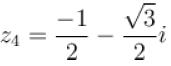*Answer can only contain numeric values
QUESTION: 7

If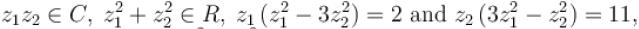then find the value of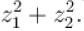Solution:

Here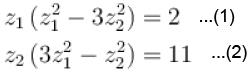multiplying (2) by i and adding it to (1) we get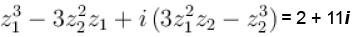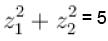*Answer can only contain numeric values
QUESTION: 8

If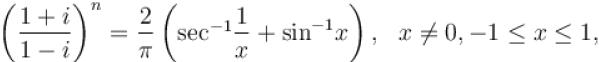then find the number of positive integers less than 20 satisfying above equation.

Solution: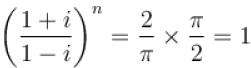Now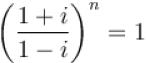⇒ r = 1
⇒ n =  4, 8, 12, 16

*Answer can only contain numeric values
QUESTION: 9

Sum of common roots of the equations z3 + 2z2 + 2z + 1 = 0  and  z97 + z29 + 1 = 0  is equal to :

Solution:

z3 + 2z2 + 2z + 1 = (z3 + 1) + 2z(z + 1)
= (z + 1)(z2 + z + 1) = 0
⇒ z = –1, ω, ω2
–1  does not satisfy  z97 + z29 + 1 = 0
but  ω and ω2 satisfy and ω + ω2 = –1
Let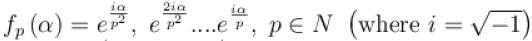, then find the value of